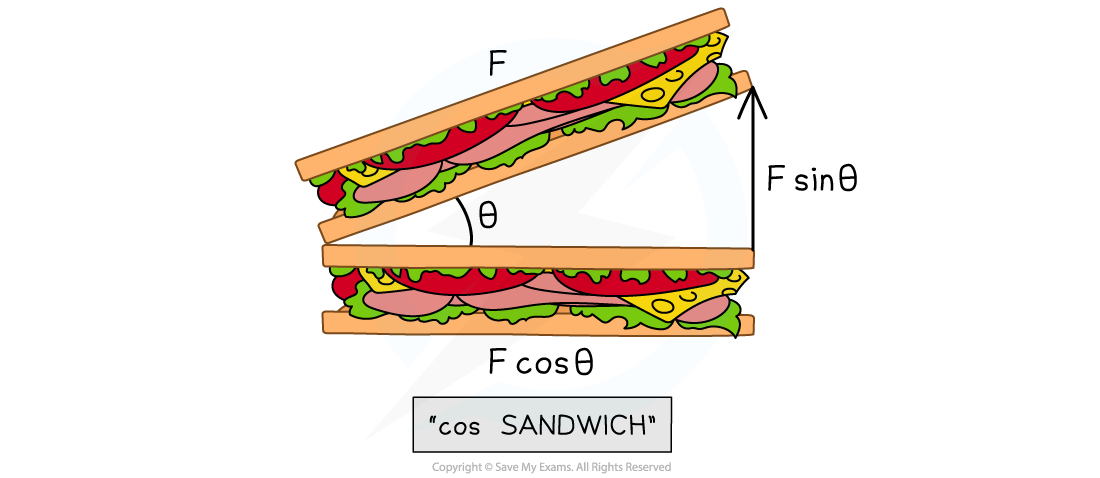# IB DP Physics: SL复习笔记1.3.3 Solving Vector Problems

### Solving Vector Problems

• In physics, vectors appear in many different topic areas
• Specifically, vectors are often combined and resolved to solve problems when considering motion, forces, and momentum

#### Forces on an Inclined Plane

• Objects on an inclined plane is a common scenario in which vectors need to be resolved
• An inclined plane, or a slope, is a flat surface tilted at an angle, θ
• Instead of thinking of the component of the forces as horizontal and vertical, it is easier to think of them as parallel or perpendicular to the slope
• The weight of the object is vertically downwards and the normal (or reaction) force, R is always vertically up from the object
• The weight W is a vector and can be split into the following components:
• W cos (θ) perpendicular to the slope
• W sin (θ) parallel to the slope
• If there is no friction, the force W sin (θ) causes the object to move down the slope
• If the object is not moving perpendicular to the slope, the normal force will be R = W cos (θ)The weight vector of an object on an inclined plane can be split into its components parallel and perpendicular to the slope

#### Worked Example

A helicopter provides a lift of 250 kN when the blades are tilted at 15º from the vertical.Calculate the horizontal and vertical components of the lift force.

Step 1: Draw a vector triangle of the resolved forces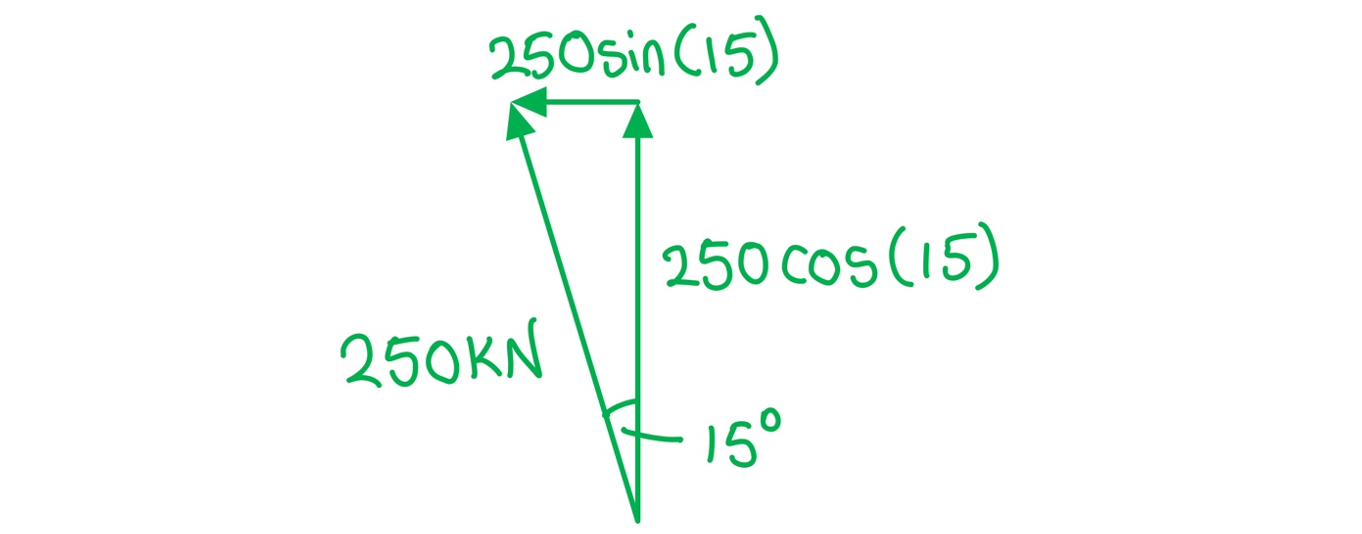Step 2: Calculate the vertical component of the lift force

Vertical = 250 × cos(15) = 242 kN

Step 3: Calculate the horizontal component of the lift force

Horizontal = 250 × sin(15) = 64.7 kN

#### Worked Example

A person is exploring a new part of town, from their starting point they walk 100 m in the direction 30.0º South of West. They then walk 200 m in the direction 40.0º degrees South of East and finally they walk 150 m directly East.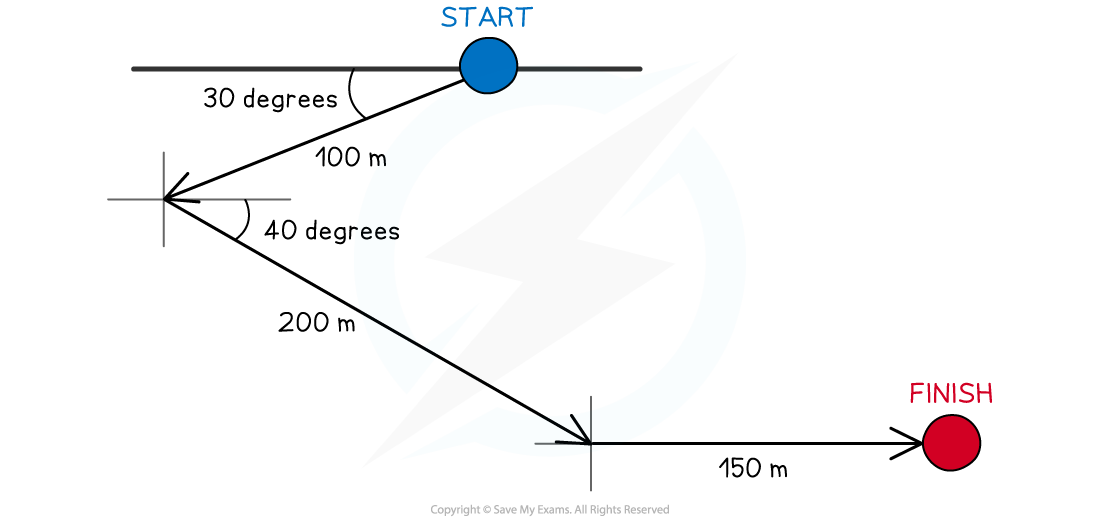Calculate the magnitude of their displacement from their original position.

In order to calculate the answer, the vectors of displacement must be resolved into their x-components and y-components and then combined. In this case, this effectively means the x-direction is East-West and the y-direction is North-South

Step 1: Consider positive and negative directions for reference

• Since East is likely to be larger consider it the positive displacement and West as negative
• Similarly, consider South as positive and North as negative

Step 2: Resolve the first displacement (100 m magnitude) into its components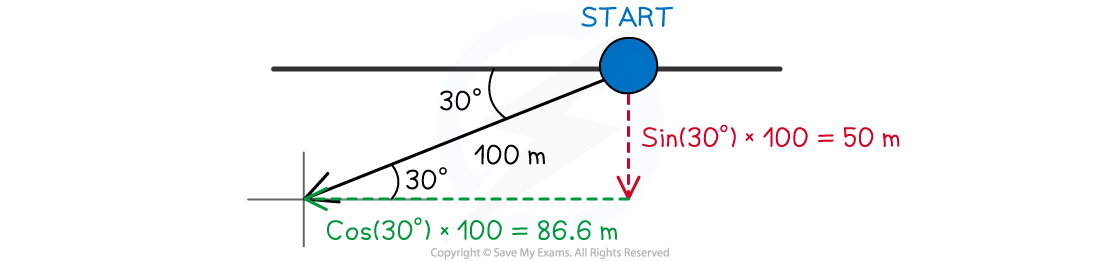• The horizontal component can be resolved from:

cos(30°) × 100 = 86.6 m

• This is in a Western (negative horizontal) direction
• The vertical component can be resolved from:

sin(30°) × 100 = 50.0 m

• This is in a Southern (positive vertical) direction

Step 3: Resolve the second displacement (200 m magnitude) into its components• The horizontal component can be resolved from:

cos(40°) × 200 = 153 m

• This is in an Eastern (positive horizontal) direction
• The vertical component can be resolved from:

sin(40°) × 200 = 129 m

• This is in a Southern (positive vertical) direction

Step 4: Resolve the third displacement (150 m magnitude) into its components

• The horizontal component is already resolved into

150 m

• This is in an Eastern (positive horizontal) direction
• There is no vertical component for this vector

Step 5: Combine the horizontal (East-West) components153 + 150 - 86.6 = 166 m

• This is in an Eastern (positive horizontal) direction

Step 6: Combine the vertical (North-South) components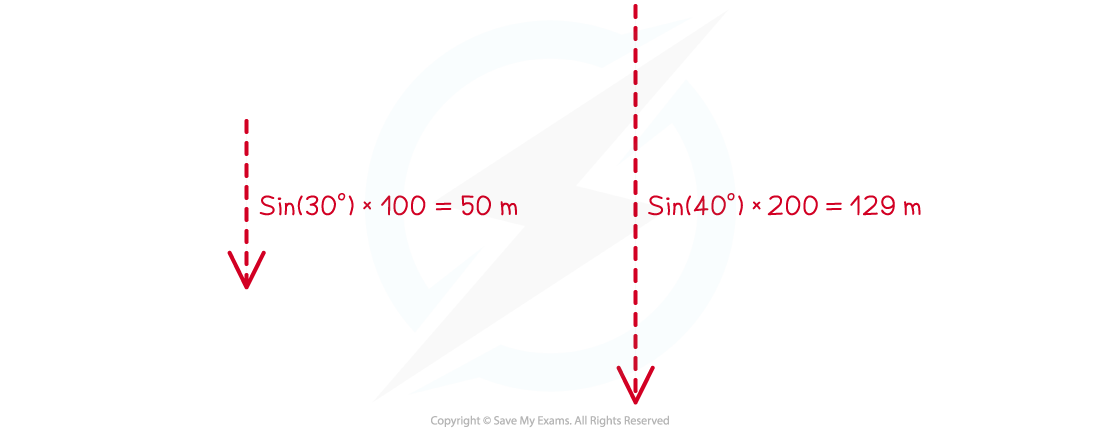50.0 + 129 = 170 m

• This is in a Southern (positive vertical) direction

Step 7: Using Pythagoras theorem to find the resultant hypotenuse vector

√(1662 + 1792) = 244 m

#### Equilibrium

• Coplanar forces can be represented by vector triangles
• Forces are in equilibrium if an object is either
• At rest
• Moving at constant velocity
• In equilibrium, coplanar forces are represented by closed vector triangles
• The vectors, when joined together, form a closed path
• The most common forces on objects are
• Weight
• Normal reaction force
• Tension (from cords and strings)
• Friction
• The forces on a body in equilibrium are demonstrated below: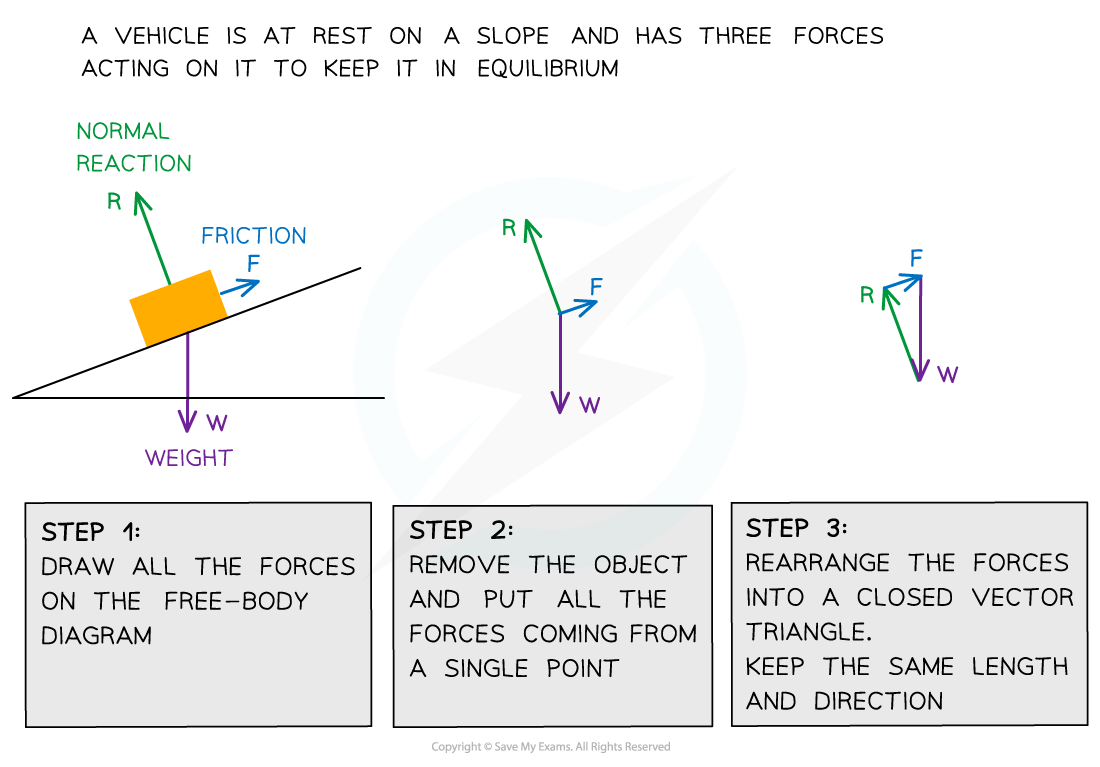Three forces on an object in equilibrium form a closed vector triangle

#### Worked Example

A weight hangs in equilibrium from a cable at point X. The tensions in the cables are T1 and T2 as shown.Which diagram correctly represents the forces acting at point X?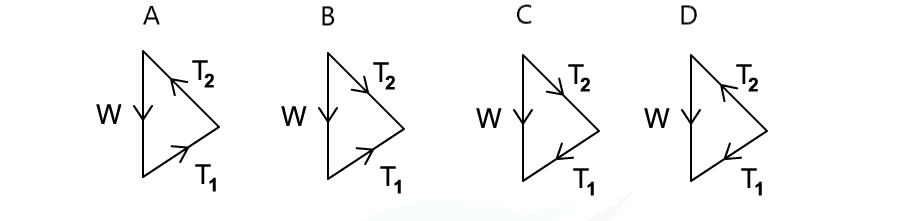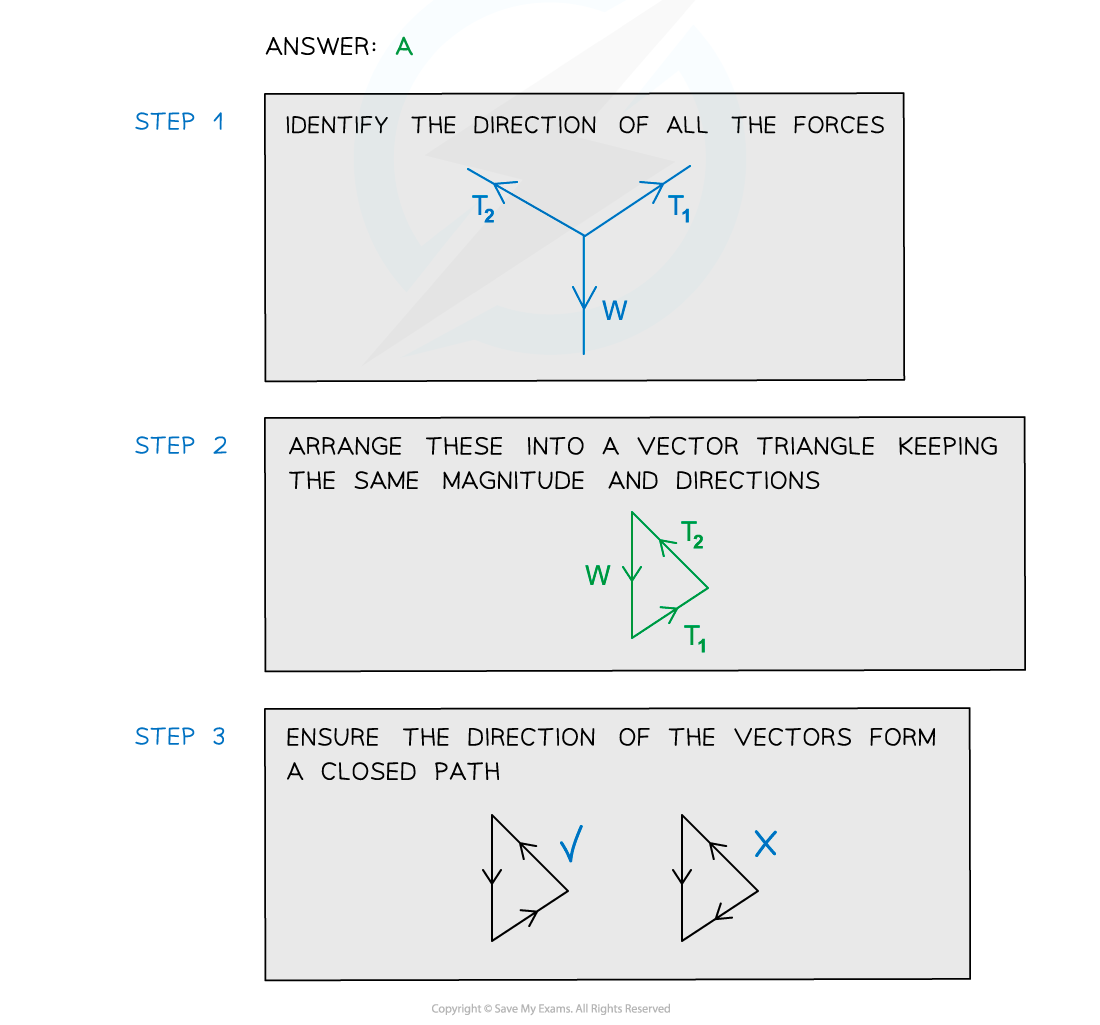#### Exam Tip

If you're unsure as to which component of the force is cos θ or sin θ, just remember that the cos θ is always the adjacent side of the right-angled triangle AKA, making a 'cos sandwich'